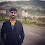### Shell Script to Create a Simple Calculator - The Coding Shala

Home >> Scripting >> simple calculator

## Shell Script to Create a Simple Calculator

Write a shell script to create a simple calculator using a switch-case statement?

Code:

```#!/bin/bash
echo "simple calculator"
sum=0
i="y"
echo "enter first number"
echo "enter second number"
while [ \$i = "y" ]
do
echo "2.Subtraction"
echo "3.Multiplication"
echo "4.Division"
echo "Enter choice"
case \$ch in
1)sum=\$(echo " \$n1 + \$n2" | bc -l)
2)sum=\$(echo "\$n1 - \$n2" | bc -l)
echo "Sub is =" \$sum;;
3)sum=\$(echo "\$n1 * \$n2" | bc -l)
echo "Mul is =" \$sum;;
4)sum=\$(echo "\$n1 / \$n2" | bc -l)
echo "div is =" \$sum;;
*)echo "invalid choice"
esac
echo "Do you want to continue"
if [ \$i != "y" ]
then
exit
fi
```

Other Posts You May Like

1.in the program when we opt to continue its taking the same number as we had given in the starting and it is asking for only operations.....we are not getting the chance to give new inputss

1.take number inputs inside the while loop.